## Capacitance of Three Phase Transmission Line with Equilateral Spacing:

Figure 3.5 shows a Capacitance of Three Phase Transmission Line with Equilateral Spacing composed of three identical conductors of radius r placed in equilateral configuration.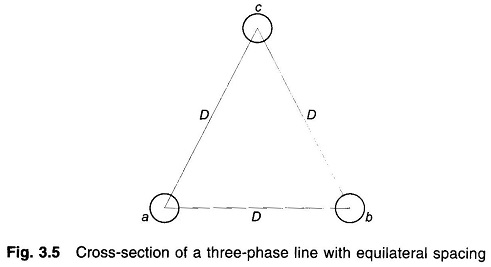Using Eq. (3.2) we can write the expressions for Vab and Vac as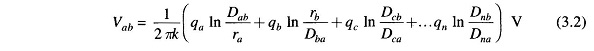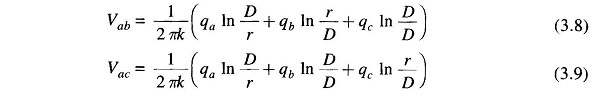Adding Eqs. (3.8) and (3 9), we get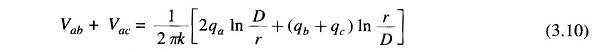Since there are no other charges in the vicinity, the sum of charges on the three conductors is zero. Thus qb + qc = – qa, which when substituted in Eq. (3.10) yields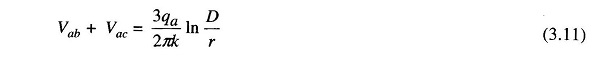With balanced three-phase voltages applied to the line, it follows from the phasor diagram of Fig. 3.6 that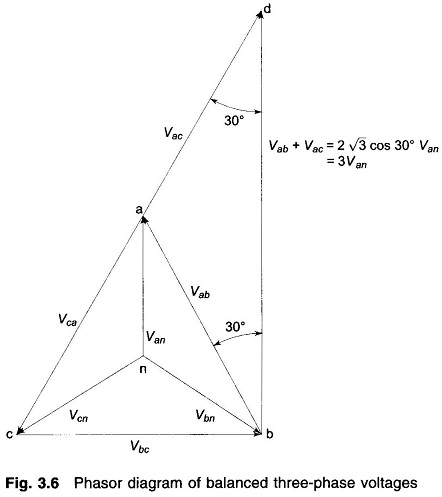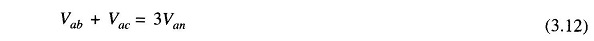Substituting for (Vab + Vac) from Eq. (3.12) in Eq. (3.11), we get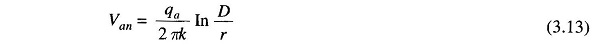The capacitance of line to neutral immediately follows as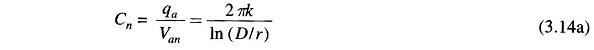For air medium (kr = 1),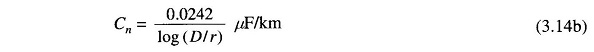The line charging current of phase a is

Scroll to Top